## 2013年4月30日 星期二

### [ Alg info ] Kruskal’s algorithm (MST)

Preface:
Kruskal 演算法是一種用來尋找 MST(minimum spanning tree) 的演算法，由 Joseph Kruskal 在1956年發表。用來解決同樣問題的還有 Prim演算法 和 Boruvka演算法等。三種演算法都是 貪婪演算法 的應用。和 Boruvka演算法 不同的地方是，Kruskal演算法 在圖中存在相同權值的邊時也有效.

Description:

1. GENERIC-MST(G,w)
2. while A does not form a spanning tree
3.     find an edge (u,v) that is safe for A
4.     A=A⋃{(u,v)}
5. return A

* A 用來存放 MST edge 的 set.
* "find an edge (u,v) that is safe for A" 指的是加上該 edge 後, 不會讓 A 中的 forest 形成 loop (acyclic).
* "A=A⋃{(u,v)}" 指把 edge (u,v) 加到 A 中.

* A 是最終的 MST 的edge的subset
* 隨時 A 都保持acyclic (No cycle)
* 每次都選一個edge, 把 A 形成的 graph 中兩棵 tree 連接起來 (因為不能出現cycle)
* 最後共選|V|-1條邊

Kruskal’s algorithm:

* MAKE-SET(v): 創造一個set 包含 v.
* FIND-SET(v): 找到set 包含 v.
* UNION(u,v): 把兩個set合併起來

1. MST-KRUSKAL(G,w)
2. A={}
3. for each vertex v∈G.V
4.     MAKE-SET(v)
5. sort G.E by w
6. for each edge (u,v) ∈G.E
7.     if FIND-SET(u)≠FIND-SET(v)
8.         A=A⋃{(u,v)}
9.         UNION(u,v)
10. return A

1. 初始時, 每個 vertex 各自屬於一個 set
2. 接著對 Graph 的 edge's weight 進行 sorting (從小到大)
3. Loop edges
3.1 如果該 edge(u,v) 的 u 與 v 分屬不同的 set, 則將該 edge 加到 A
3.2 將分屬於 u 與 v 的 set 進行 union.

Example: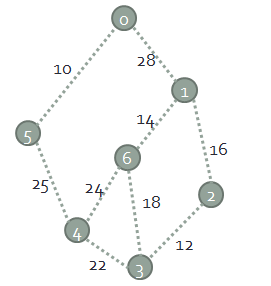1. Sorting 過後, 第一個取出來的 edge(0,5). 因為 vertex 0, 5 分屬不同的 set, 故將此 edge 加到 A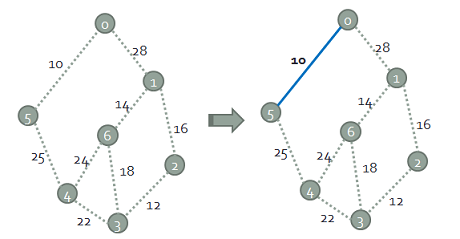2. 接著第二個取出來的 edge(2,3). 因為 vertex 2, 3 分屬不同的 set, 故將此 edge 加到 A3. 接著第三個取出來的 edge(1,6). 因為 vertex 1, 6 分屬不同的 set, 故將此 edge 加到 A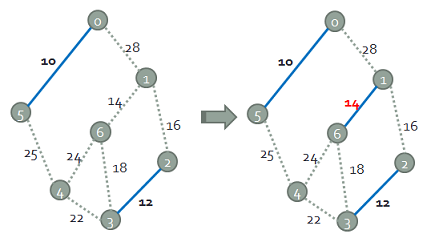4. 接著第四個取出來的 edge(1,2). 因為 vertex 1, 2 分屬不同的 set, 故將此 edge 加到 A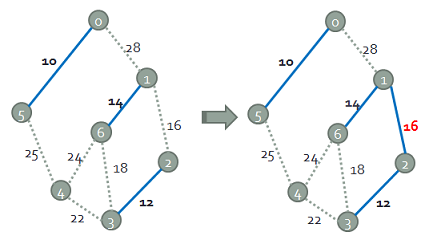5. 接著第五個取出來的 edge(3,6). 因為 vertex 3, 6 屬於同一個 set {(1,2), (1,6), (2,3)}, 故捨棄此 edge!6. 接著第六個取出來的 edge(3,4). 因為 vertex 3, 4 分屬不同的 set, 故將此 edge 加到 A7. 接著第七個取出來的 edge(4,6). 因為 vertex 4, 6 屬於同一個 set {(1,2), (1,6), (2,3), (3,4)}, 故捨棄此 edge!8. 接著第八個取出來的 edge(4,5). 因為 vertex 4, 5 分屬不同的 set, 故將此 edge 加到 A; 且此時 A 有 6 個 edges = |V|-1. 故 MST 已經完成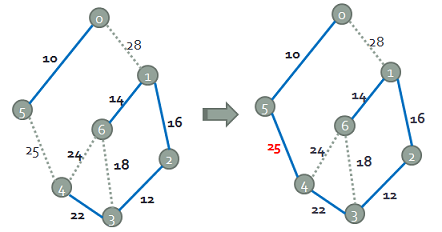Supplement:
[ Alg info ] Borůvka's algorithm (MST)
[ Data Structures with Java ] Section 25.6 : Minimum Spanning Tree
[ 資料結構 小學堂 ] 圖形結構 : 擴張樹 (Prim)
[ 資料結構 小學堂 ] 圖形結構 : 擴張樹 - 求最小成本擴張樹 (Kruskal 演算法)

### [ Py DS ] Ch5 - Machine Learning (Part2)

Source From  Here   Introducing Scikit-Learn   There are several Python libraries that provide solid implementations of a range of machin...